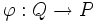# Weakly closed subgroup for a fusion system

This article defines a property that can be evaluated for a group of prime power order, equipped with a fusion system
View other such properties

## Definition

Suppose$P$ is a group of prime power order and$\mathcal{F}$ is a fusion system on$P$. Then a subgroup$Q \le P$ is termed weakly closed with respect to$\mathcal{F}$ if for every morphism$\varphi: Q \to P$ in$\mathcal{F}$, we have$\varphi(Q) = Q$.

## Relation with other properties

### Related subgroup properties and subgroup-of-subgroup properties

• Weakly closed subgroup: Suppose$H \le K \le G$ are groups.$H$ is weakly closed in$K$ with respect to$G$ if, for any$g \in G$,$gHg^{-1} \le K$ implies that$gHg^{-1} \le H$.
• Weakly closed subgroup of Sylow subgroup: The case where$K$ is a$p$-Sylow subgroup of$G$. This is related to fusion systems as follows: a subgroup of a$p$-Sylow subgroup$K$ of a finite group$G$ is weakly closed in$K$ if and only if it is weakly closed for the fusion system induced by$G$ on$K$.
• Sylow-relatively weakly closed subgroup: A subgroup of a group of prime power order that is a weakly closed subgroup in any group containing the bigger group as a Sylow subgroup.
• Fusion system-relatively weakly closed subgroup: A subgroup of a group of prime power order that is weakly closed in the fusion system sense).for any fusion system on the bigger group.